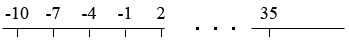SEARCH HOMEMath Central Quandaries & QueriesQuestion from Ally: -10 ... last term 35 common difference is 3 (a) find the number of term in the sequence. i have lost the formula.Hi,

I don't remember formulas either but sine you mention the common difference is 3 I know this is an arithmetic sequence. The sequence starts at -10 and extends to 35. That's a distance of 45 units.Suppose you are walking on the number line starting at -10, taking steps of length 3 units, and stopping at 35.

How many steps did you take?
How many terms are there in the sequence?

Be careful! The two questions above do not have the same answer,
PennyMath Central is supported by the University of Regina and The Pacific Institute for the Mathematical Sciences.